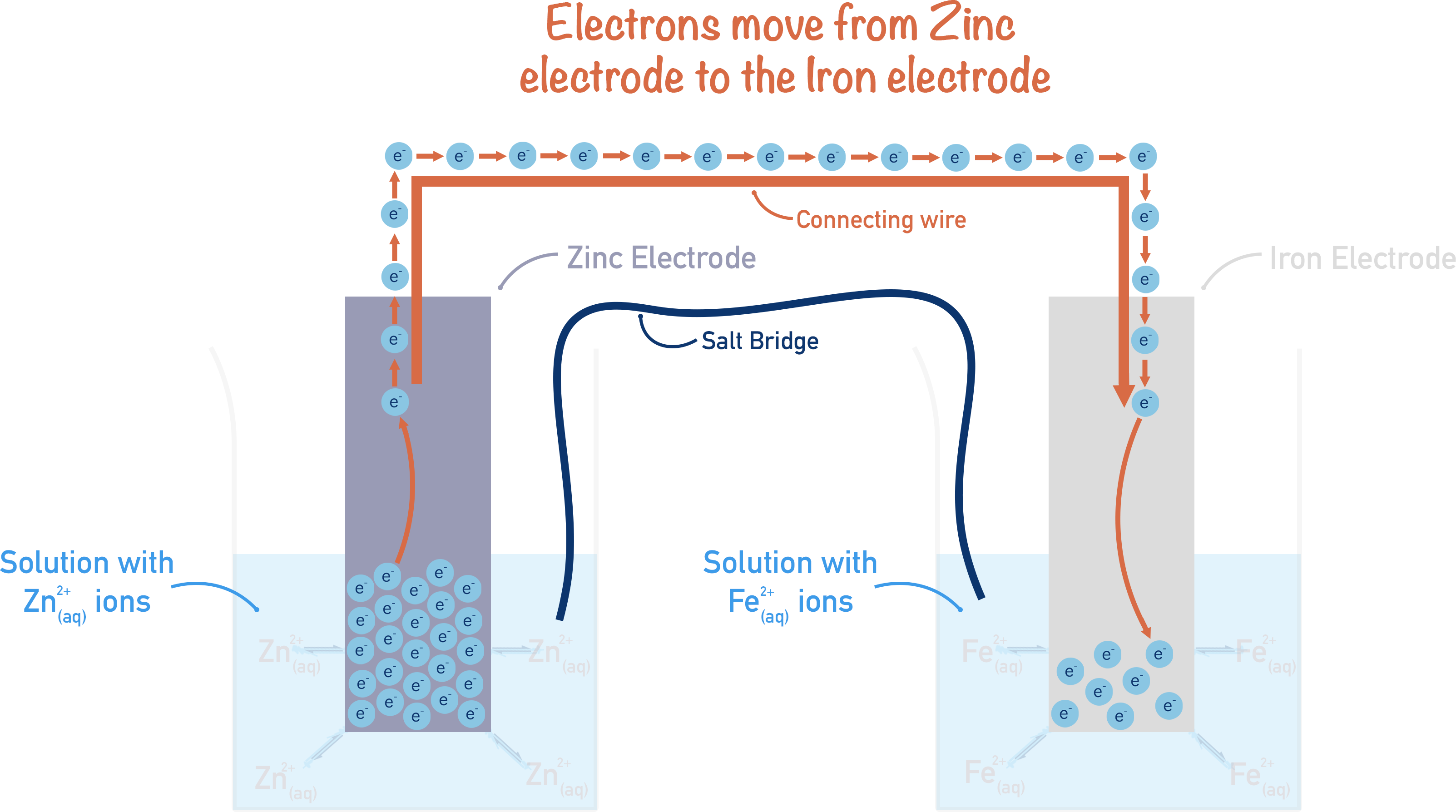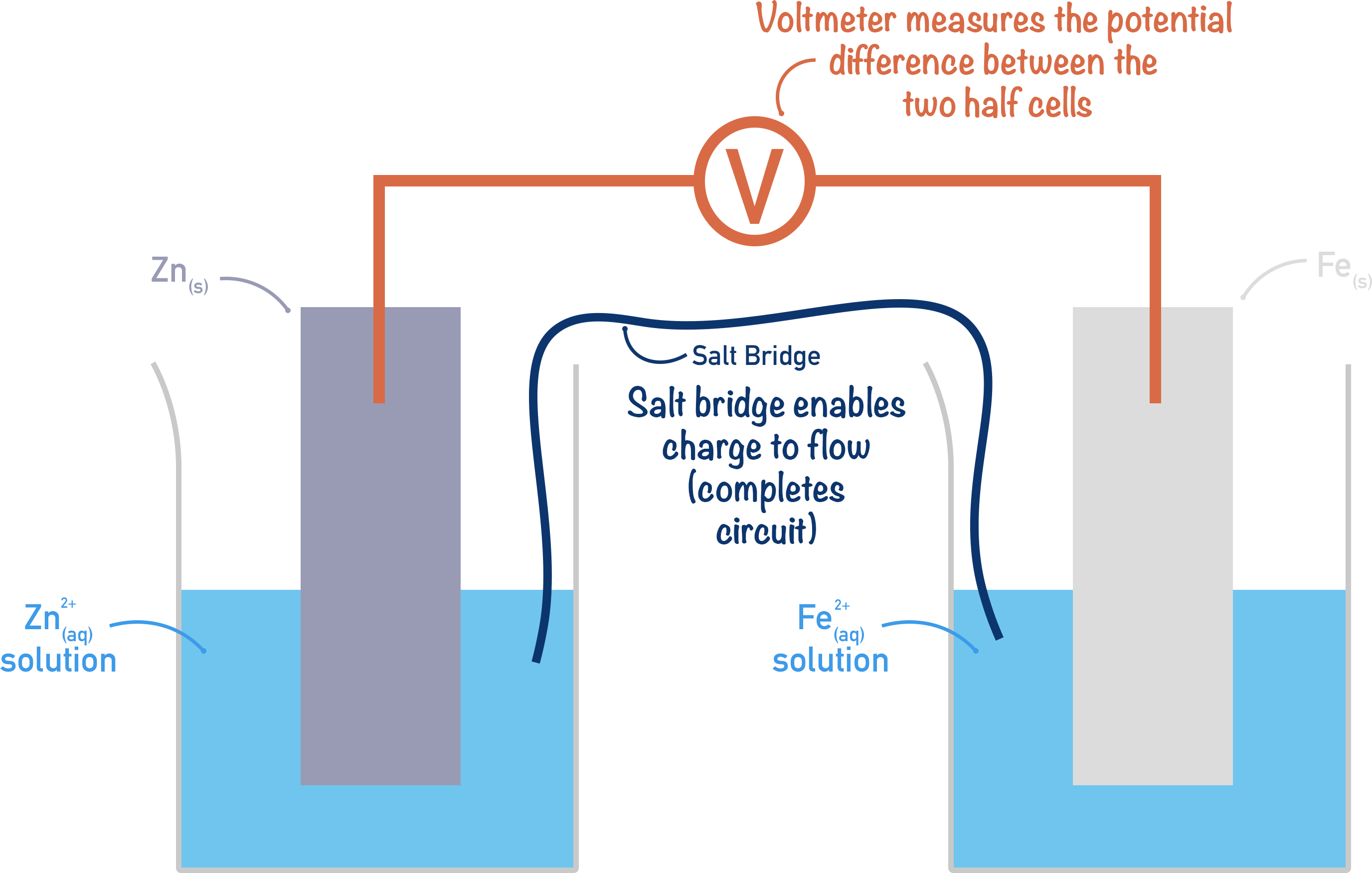Quick Notes Electrochemical Cells

• If two different half-cells are connected by a wire (and a salt bridge), an electrical current is created and electricity flows.
• This is called an electrochemical cell.
• The half-cell with the most electrons on its electrode (more negative electrode potential) will force its electrons to flow to the half-cell with fewer electrons on its electrode (more positive electrode potential).
• The potential difference between the two connected electrodes can be measured using a voltmeter.
• The greater the difference in potential (voltage) between the two half-cells, the greater the electrical current produced when they are connected.
• Electrical current is the net movement of charge in a direction.
• Electrons have a charge, so if there’s a net movement of electrons in one direction, an electrical current is produced.
• It’s the relative difference between potentials that’s important in electrochemistry.
• It’s not possible to measure the actual potential of a half-cell, just how different it is compared to other half-cells.

Full Notes Electrochemical Cells

Two different half-cells connected together form an electrochemical cell.

When two different half-cells are combined, interesting things can happen!

Let’s take a zinc half-cell and an iron half-cell.

We know that zinc is more reactive than iron, so the zinc (electrode) will have a greater number of electrons on its surface than the iron (electrode). This is because the position of equilibrium for the Zn2+ / Zn reaction lies more to the left than with the Fe2+ / Fe reaction. .

If these two half-cells were connected by a wire, electrons would flow from where they are highest in number (zinc electrode) to where they are lowest in number (iron electrode). Or, in other words, from the half-cell with the more negative electrode potential to the half-cell with the more positive electrode potential.Note, for charge to flow a circuit has to be complete, so a salt bridge is used between the half-cells. A salt bridge is simply made from paper or material soaked in a salt solution. The salt solution enables charged ions to flow between the half-cells, completing a circuit for electrical current to flow. It’s important to note that the salt used must contain ions that won’t interact with the half-cell reactions!

Key physics point! Electricity is the net movement of charge in one direction. If you have charged particles moving in one direction, you have an electrical current (‘electricity’).

In the above example, a small electrical current is formed because electrons move from the zinc half-cell to the iron half-cell through the connecting wire. The movement of electrons occurs because there is a difference in the electrode potentials of each half-cell; this is what ‘produces’ an electrical current (electricity).

The amount of current produced is a result of how much the electrode potentials of each half-cell differ (hence, potential difference – the difference in two potentials).

If there is a big potential difference between two connected half-cells, a large current flows between them; if there is a small potential difference between two connected half-cells, a small current flows between them. The potential difference between two half-cells can be measured using a voltmeter connected between them.

Note, the voltmeter used to measure the potential difference must have a very high ‘resistance’ to ensure no current can flow through it. Potential difference is measured in volts (V).The key thing here is that the potential difference of an electrochemical cell is based on the half-cells used and their relative electrode potentials.

If one half-cell has an electrode potential of 10V and the other 11V, the potential difference of the electrochemical cell would be 1V. Equally, if one half-cell has a potential of 0.1V and the other 1.1V, the potential difference of the whole cell would still be 1V.

All this means that we are only interested in the electrode potentials of each half-cell relative to other half-cells. The actual potential of a half-cell is largely meaningless in electrochemistry, we need to know how one half-cell compares to another.

To overcome this, different half-cells are all connected up to the same half-cell, and the potential difference of the electrochemical cell is measured. This gives a table of measurements that can be used to help compare the electrode potentials of different half-cells, without actually having to connect them together.

The same half-cell used each time is the standard hydrogen electrode and the measurements made are called standard electrode potentials. .